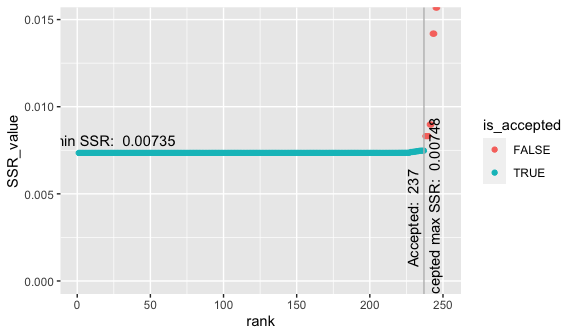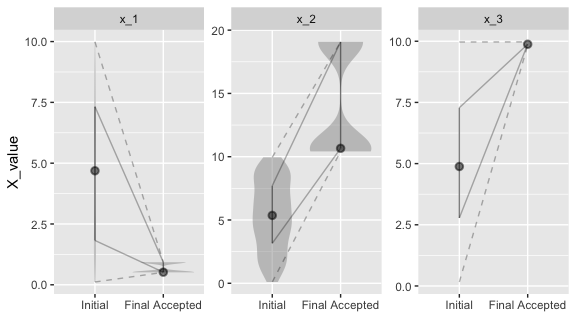# CGNM: Cluster Gauss-Newton Method

library(CGNM)

# When and when not to use CGNM

## Use CGNM

• Wish to fit relatively complex parameterized model/curve/function to the data; however, unsure of the appropriate initial guess (e.g., “start” argument in nls function). => CGNM searches the parameter from multiple initial guess so that the use can specify rough initial range instad of a point initial guess.
• When the practical identifiability (if there is only one best fit parameter or not) is unknown. => CGNM will find multiple set of best fit parameters; hence if the parameter is not practically identifiable then the multuple best-fit parameters found by CGNM will not converge to a point.
• When the model is a blackbox (i.e., cannot be expricitly/easily write out as a “formula”) and the model may not be continuous with respect to the parameter. => CGNM makes minimum assumptions on the model so all user need to provide is a function that takes the model parameter and simulation (and what happen in the function, CGNM does not care).

## Not to use CGNM

• When the you already know where approximately the best fit parameter is and you just need to find one best fit parameter. => Simply use nls and that will be faster.
• When the model is relatively simple and computation cost is not an issue. => CGNM is made to save the number of model evaluation dueing the parameter estimation, so may not see much advantage compared to the conventional multi-start methods (e.g.repeatedly using nls from various “start”).

# How to use CGNM

To illutrate the use of CGNM here we illustrate how CGNM can be used to estiamte two sets of the best fit parameters of the pharmacokinetics model when the drug is administered orally (known as flip-flop kinetics). To illustrate that the model can be definied flexibly, we use the RxODE package to define the model.

## Prepare the model ($$\boldsymbol f$$)

library(RxODE)
#>   no cache: create with rxCreateCache()
#> ========================================
#> RxODE has not detected OpenMP support and will run in single-threaded mode
#> ========================================

model_text="
d/dt(X_1)=-ka*X_1
d/dt(C_2)=(ka*X_1-CL_2*C_2)/V1"

# here the model defined as above is compiled
model=RxODE(model_text)

# here we define the model function where takes in the parameter vector x and return the model simulation
model_function=function(x){

observation_time=c(0.1,0.2,0.4,0.6,1,2,3,6,12)

theta <- c(ka=x,V1=x,CL_2=x)
ev <- eventTable()
ev$add.dosing(dose = 1000, start.time =0) ev$add.sampling(observation_time)
odeSol=model\$solve(theta, ev)
log10(odeSol[,"C_2"])
}

## Prepare the data ($$\boldsymbol y^*$$)

observation=log10(c(4.91, 8.65, 12.4, 18.7, 24.3, 24.5, 18.4, 4.66, 0.238))

## Run Cluster_Gauss_Newton_method

Here we have specified the upper and lower range of the initial guess.

CGNM_result=Cluster_Gauss_Newton_method(nonlinearFunction=model_function,
targetVector = observation,
initial_lowerRange = c(0.1,0.1,0.1),initial_upperRange =  c(10,10,10))
#>  "checking if the nonlinearFunction can be evaluated at the initial_lowerRange"
#>  "Evaluation Successful"
#>  "checking if the nonlinearFunction can be evaluated at the initial_upperRange"
#>  "Evaluation Successful"
#>  "checking if the nonlinearFunction can be evaluated at the (initial_upperRange+initial_lowerRange)/2"
#>  "Evaluation Successful"
#>  "Generating initial cluster. 196 out of 250 done"
#>  "Generating initial cluster. 241 out of 250 done"
#>  "Generating initial cluster. 248 out of 250 done"
#>  "Generating initial cluster. 250 out of 250 done"
#>  "Iteration:1  Median sum of squares residual=4.77275971346861"
#>  "Iteration:2  Median sum of squares residual=2.71946046697338"
#>  "Iteration:3  Median sum of squares residual=0.884225581108638"
#>  "Iteration:4  Median sum of squares residual=0.67850930826005"
#>  "Iteration:5  Median sum of squares residual=0.173641035795561"
#>  "Iteration:6  Median sum of squares residual=0.0122631832161518"
#>  "Iteration:7  Median sum of squares residual=0.00741113112121069"
#>  "Iteration:8  Median sum of squares residual=0.00734930088163792"
#>  "Iteration:9  Median sum of squares residual=0.00734926992791461"
#>  "Iteration:10  Median sum of squares residual=0.00734926727781488"
#>  "Iteration:11  Median sum of squares residual=0.00734926725997317"
#>  "Iteration:12  Median sum of squares residual=0.0073492672328896"
#>  "Iteration:13  Median sum of squares residual=0.00734926720919953"
#>  "Iteration:14  Median sum of squares residual=0.00734926720847175"
#>  "Iteration:15  Median sum of squares residual=0.00734926720334394"
#>  "Iteration:16  Median sum of squares residual=0.00734926718148023"
#>  "Iteration:17  Median sum of squares residual=0.00734926718148023"
#>  "Iteration:18  Median sum of squares residual=0.00734926718148023"
#>  "Iteration:19  Median sum of squares residual=0.0073492671786963"
#>  "Iteration:20  Median sum of squares residual=0.00734926717743428"
#>  "Iteration:21  Median sum of squares residual=0.00734926717743428"
#>  "Iteration:22  Median sum of squares residual=0.00734926717310454"
#>  "Iteration:23  Median sum of squares residual=0.00734926717249444"
#>  "Iteration:24  Median sum of squares residual=0.00734926716012509"
#>  "Iteration:25  Median sum of squares residual=0.00734926715183214"

## Obtain the approximate minimizers

head(acceptedApproximateMinimizers(CGNM_result))
#>           [,1]     [,2]     [,3]
#> [1,] 0.5178937 10.66078 9.877358
#> [2,] 0.9265012 19.07198 9.877309
#> [3,] 0.9265246 19.07234 9.877390
#> [4,] 0.5178937 10.66081 9.877339
#> [5,] 0.5178959 10.66084 9.877311
#> [6,] 0.5178979 10.66100 9.877236

## Visualize the distributions of the parameters found by CGNM

To use the plot functions the user needs to manually load ggplot2.

library(ggplot2)
#>
#> Attaching package: 'ggplot2'
#> The following object is masked from 'package:RxODE':
#>
#>     facet_wrap
plot_Rank_SSR(CGNM_result)plot_paraDistribution_byViolinPlots(CGNM_result)# What is CGNM?

For the complete description and comparison with the conventional algorithm please see (https: //doi.org/10.1007/s11081-020-09571-2):

Aoki, Y., Hayami, K., Toshimoto, K., & Sugiyama, Y. (2020). Cluster Gauss–Newton method. Optimization and Engineering, 1-31.

## The mathematical problem CGNM solves

Cluster Gauss-Newton method is an algorithm for obtaining multiple minimisers of nonlinear least squares problems $\min_{\boldsymbol{x}}|| \boldsymbol{f}(\boldsymbol x)-\boldsymbol{y}^*||_2^{\,2}$ which do not have a unique solution (global minimiser), that is to say, there exist $$\boldsymbol x^{(1)}\neq\boldsymbol x^{(2)}$$ such that $\min_{\boldsymbol{x}}|| \boldsymbol{f}(\boldsymbol x)-\boldsymbol{y}^*||_2^{\,2}=|| \boldsymbol{f}(\boldsymbol x^{(1)})-\boldsymbol{y}^*||_2^{\,2}=|| \boldsymbol{f}(\boldsymbol x^{(2)})-\boldsymbol{y}^*||_2^{\,2} \,.$ Parameter estimation problems of mathematical models can often be formulated as nonlinear least squares problems. Typically these problems are solved numerically using iterative methods. The local minimiser obtained using these iterative methods usually depends on the choice of the initial iterate. Thus, the estimated parameter and subsequent analyses using it depend on the choice of the initial iterate. One way to reduce the analysis bias due to the choice of the initial iterate is to repeat the algorithm from multiple initial iterates (i.e. use a multi-start method). However, the procedure can be computationally intensive and is not always used in practice. To overcome this problem, we propose the Cluster Gauss-Newton method (CGNM), an efficient algorithm for finding multiple approximate minimisers of nonlinear-least squares problems. CGN simultaneously solves the nonlinear least squares problem from multiple initial iterates. Then, CGNM iteratively improves the approximations from these initial iterates similarly to the Gauss-Newton method. However, it uses a global linear approximation instead of the Jacobian. The global linear approximations are computed collectively among all the iterates to minimise the computational cost associated with the evaluation of the mathematical model.